## Abstract

An analysis of thermal boundary layer in the flow of Casson fluid over a permeable shrinking sheet with variable wall temperature and thermal radiation is made. Using similarity transformations, self-similar nonlinear ODEs are obtained from the governing equations. Dual exact solutions of transformed velocity and energy equations are obtained. From the plotted results it can be observed that the temperature inside the boundary layer decreases with Casson parameter and wall mass transfer parameter in first solution and it increases in second solution. Whereas, temperature decreases for larger values of Prandtl number, radiation parameter and power-law exponent for inverse variation along the sheet in both solutions and it enhances with power-law exponent for direct variation along the surface. Also, thermal boundary layer thickness reduces with stronger thermal radiation and inverse variation of wall temperature along the surface and it becomes thicker with direct variation of wall temperature. The rate of heat transfer is less with increasing values of power-law exponent for direct variation along the sheet and for inverse variation it is higher. In graphical representation of temperature field, temperature overshoot is observed in certain cases. So, in some situations heat absorption at surface occurs instead of heat transfer from surface.

## Keywords

Thermal boundary layer; Casson fluid; Shrinking sheet; Exact dual solutions; Variable wall temperature; Thermal radiation

## 1. Introduction

The boundary layer flow due to a shrinking sheet is emerged as an interesting problem in fluid dynamics. The shrinking sheet flows occur in some practical situations, such as, for rising shrinking balloon and it is very useful in packaging of bulk products. The heat transfer plays a vital role in the flow due to shrinking. Miklavčič and Wang  demonstrated that the steady flow of Newtonian fluid over a shrinking sheet is possible only when the adequate amount of mass suction through the porous sheet is applied. Actually, the mass suction suppresses the generated vorticity due to shrinking inside the boundary layer and maintains boundary layer flow. Later, Fang and Zhang  explained the influence of external magnetic field on the shrinking sheet flow and found that the strong magnetic field guarantees the steady boundary layer flow. Thereafter, the Newtonian fluid flow past a shrinking sheet is investigated by many researchers , , , , , , , , , , ,  and  under various physical aspects.

On the other hand, Hayat et al. ,  and  discussed the non-Newtonian fluid flows over a shrinking sheet. In reality, the majority of the fluids appeared in the technological processes shows non-Newtonian fluid properties , , , , ,  and . So, the studies of non-Newtonian flows are very crucial in technological point of view. Some significant investigations on the shrinking sheet flow of non-Newtonian fluids were reported by Ishak et al.  and , Yacob et al.  and  and Rosali et al. . Later, Nadeem et al.  investigated MHD flow of a Casson fluid over an exponentially shrinking sheet. Recently, Bhattacharyya et al.  and  discussed the flow dynamics of Casson fluid over stretching/shrinking sheet with and without effect of magnetic field.

The thermal radiation effect on the boundary layer flow is relevant in many engineering problems because of its applications especially in high temperature engineering processes. Heat radiation effect is important in controlling the quality of the final product as it affects the rate cooling. Due to the above fact, important works on thermal radiation effect had been done by some researchers, viz., Hossain and Takhar , Elbashbeshy , Mukhopadhyay , Mukhopadhyay et al.  Akbar et al.  and Bhattacharyya . The radiation effects on the boundary layer flow of Newtonian fluid and heat transfer over shrinking sheet were investigated by Bhattacharyya and his co-workers ,  and  and Ali et al. . The radiation effect becomes more interesting when the wall temperature distribution is variable. Pal et al.  showed the effect of thermal radiation on convection–dissipation heat transfer over stretching/shrinking sheet with variable surface temperature.

But very little is known about the simultaneous effects of radiation and variable wall temperature on the boundary layer flow of non-Newtonian fluid and heat transfer. So, the effects of thermal radiation and the variable surface temperature on the thermal boundary layer of Casson fluid flow over a shrinking sheet are still unknown. The significance of heat radiation effects on the heat flow when wall temperature is variable motivates us to study this paper. Hence, in the present paper the heat transfer in boundary layer flow of non-Newtonian Casson fluid over a shrinking sheet with variable wall temperature and thermal radiation is investigated. Similarity transformations are used and the exact solutions for velocity and temperature distributions are obtained.

## 2. Flow analysis

Consider the steady two-dimensional incompressible flow of Casson fluid bounded by a shrinking sheet at y = 0, the flow being confined in y > 0. We assume also that the rheological equation of state for an isotropic and incompressible flow of a Casson fluid can be written as follows (see Nakamura and Sawada ):

 ${\tau }_{ij}={\begin{array}{c}\left({\mu }_{B}+p_{y}/{\sqrt {2\pi }}\right)2e_{ij}{\mbox{,}}\quad \pi >{\pi }_{c}\\\left({\mu }_{B}+p_{y}/{\sqrt {2{\pi }_{c}}}\right)2e_{ij}{\mbox{,}}\quad \pi <{\pi }_{c}{\mbox{,}}\end{array}}$
(1)

where μB is plastic dynamic viscosity of the non-Newtonian fluid, py is the yield stress of fluid, π is the product of the component of deformation rate with itself, namely, π = eijeij, eij is the (i, j)-th component of the deformation rate and πc is critical value of π based on non-Newtonian model.

Under these conditions the boundary layer equations for the steady flow of Casson fluid may be written as follows :

 ${\frac {\partial u}{\partial x}}+{\frac {\partial v}{\partial y}}=$$0{\mbox{,}}$
(2)

 $u{\frac {\partial u}{\partial x}}+v{\frac {\partial u}{\partial y}}=$$\upsilon \left(1+{\frac {1}{\beta }}\right){\frac {{\partial }^{2}u}{\partial y^{2}}}{\mbox{,}}$
(3)

where u and v are the velocity components in x and y directions respectively, υ is the kinematic fluid viscosity, ρ   is the fluid density, ${\textstyle \beta ={\mu }_{B}{\sqrt {2{\pi }_{c}}}/p_{y}}$ is the non-Newtonian (Casson) parameter.

The boundary conditions for the velocity components are

 $u=-U_{w}{\mbox{,}}\quad v=-v_{w}\quad \quad {\mbox{at}}\quad \quad y=$$0{\mbox{;}}u\rightarrow 0\quad \quad {\mbox{as}}\quad \quad y\rightarrow \infty {\mbox{,}}$
(4)

where Uw = cx is shrinking velocity of the sheet with c(>0) being the shrinking constant. Here vw is the wall mass transfer velocity with vw > 0 for mass suction and vw < 0 for mass injection.

The following similarity transformations are introduced:

 $\psi ={\sqrt {\upsilon {xU}_{w}}}\quad f(\eta )\quad {\mbox{and}}\quad \eta =$$y{\sqrt {\frac {U_{w}}{\upsilon x}}}{\mbox{,}}$
(5)

where ψ   is the stream function defined in the usual notation as ${\textstyle u={\frac {\partial \psi }{\partial y}}\quad \quad {\mbox{and}}\quad \quad v=}$$-{\frac {\partial \psi }{\partial x}}$ and η is the similarity variable.

In view of the relations in (5), the Eq. (2) satisfies automatically and the Eq. (3) reduces to the following self-similar equation:

 $\left(1+{\frac {1}{\beta }}\right)f^{'''}+{ff}^{''}-$$f^{{'}2}=0{\mbox{,}}$
(6)

where primes denote differentiation with respect to η.

The boundary conditions become

 $f(\eta )=S{\mbox{,}}f^{'}(\eta )=-1\quad \quad {\mbox{at}}\quad \quad \eta =$$0{\mbox{;}}f^{'}(\eta )\rightarrow 0\quad \quad {\mbox{as}}\quad \quad \eta \rightarrow \infty {\mbox{,}}$
(7)

where S = vw/()1/2 is wall mass transfer parameter with S > 0 (i.e. vw > 0) for mass suction and S < 0 (i.e. vw < 0) for mass injection.

## 3. Heat transfer analysis

To know the behaviour of temperature distribution in the abovementioned flow field, the following energy equation using boundary layer approximation (neglecting viscous dissipation) needs to be solved:

 $u{\frac {\partial T}{\partial x}}+v{\frac {\partial T}{\partial y}}=$${\frac {\kappa }{\rho c_{p}}}{\frac {{\partial }^{2}T}{\partial y^{2}}}-$${\frac {1}{\rho c_{p}}}{\frac {\partial q_{r}}{\partial y}}{\mbox{,}}$
(8)

where T is the temperature, κ is the thermal conductivity, cp is the specific heat and qr is the radiative heat flux.

The appropriate boundary conditions are

 $T=T_{w}=T_{\infty }+T_{0}x^{n}\quad \quad {\mbox{at}}\quad \quad y=$$0{\mbox{;}}T\rightarrow T_{\infty }\quad \quad {\mbox{as}}\quad \quad y\rightarrow \infty {\mbox{,}}$
(9)

where Tw is the variable temperature along the sheet, T is the free stream temperature assumed to be constant, T0 is a constant depended on the thermal properties of the fluid and n is a power-law exponent.

Using Rosseland approximation for radiation , qr = −(4σ/3k1)∂T4/∂y is obtained, where σ is the Stefan–Boltzmann constant and k1 is the absorption coefficient. We presume that the temperature variation within the flow is such that T4 may be expanded in a Taylor’s series. Expanding T4 about T and neglecting higher order terms we get, T4 = 4T3T − 3T4.

Now Eq. (8) reduces to

 $u{\frac {\partial T}{\partial x}}+v{\frac {\partial T}{\partial y}}=$${\frac {\kappa }{\rho c_{p}}}{\frac {{\partial }^{2}T}{\partial y^{2}}}+$${\frac {16\sigma T_{\infty }^{3}}{3k_{1}\rho c_{p}}}{\frac {{\partial }^{2}T}{\partial y^{2}}}{\mbox{.}}$
(10)

Next, the dimensionless temperature θ is introduced as

 $\theta (\eta )={\frac {T-T_{\infty }}{T_{w}-T_{\infty }}}{\mbox{.}}$
(11)

Using the relations in (5) and (11), the Eq. (10) reduces to

 $(3R+4){\theta }^{''}+3RPr[f{\theta }^{'}-{nf}^{'}\theta ]=$$0{\mbox{,}}$
(12)

where primes denote differentiation with respect to η, Pr = cpμ/κ is the Prandtl number and R = κk1/4σT3 is the thermal radiation parameter.

The boundary conditions for θ are obtained from (9) as follows:

 $\theta (\eta )=1\quad \quad {\mbox{at}}\quad \quad \eta =$$0{\mbox{;}}\theta (\eta )\rightarrow 0\quad \quad {\mbox{as}}\quad \quad \eta \rightarrow \infty {\mbox{.}}$
(13)

## 4. Solution procedure

Exact closed form solutions of Eqs. (6) and (12) with the boundary conditions (7) and (13) will be obtained.

Let us assume that the solution of Eqs. (6) with (7) has the form ${\textstyle f(\eta )=a+{be}^{-\lambda \eta }}$, where a, b and λ(>0) are constants.

Substituting in Eqs. (6) and (7), the following is obtained:

 $b={\frac {1}{\lambda }}{\mbox{,}}\quad a=S-{\frac {1}{\lambda }}\quad \quad {\mbox{and}}\quad \quad \lambda =$${\frac {S\pm {\sqrt {S^{2}-4(1+1/\beta )}}}{2(1+1/\beta )}}{\mbox{.}}$
(14)

Now the closed form solution is obtained as

 $f(\eta )=S-{\frac {1}{\lambda }}+{\frac {1}{\lambda }}e^{-\lambda \eta }$
 ${\mbox{i}}.{\mbox{e}}.{\mbox{,}}\quad f(\eta )=S-{\frac {2(1+1/\beta )}{S\pm {\sqrt {S^{2}-4(1+1/\beta )}}}}+$${\frac {2(1+1/\beta )}{S\pm {\sqrt {S^{2}-4(1+1/\beta )}}}}e^{-{\frac {S\pm {\sqrt {S^{2}-4(1+1/\beta )}}}{2(1+1/\beta )}}\eta }{\mbox{.}}$
(15)

So, ${\textstyle f^{'}(\eta )=-e^{-{\frac {S\pm {\sqrt {S^{2}-4(1+1/\beta )}}}{2(1+1/\beta )}}\eta }}$ and ${\textstyle f^{''}(0)=\lambda ={\frac {S\pm {\sqrt {S^{2}-4(1+1/\beta )}}}{2(1+1/\beta )}}}$.

For physically feasible solution of the steady Casson fluid flow λ be positive, the steady flow is possible only if wall mass suction parameter S(>0) satisfies the following:

 $S^{2}\geqslant 4(1+1/\beta ){\mbox{.}}$
(16)

The similarity solution is unique if ${\textstyle S^{2}=4(1+1/\beta )}$, it is of dual nature if ${\textstyle S^{2}>4(1+1/\beta )}$ and no similarity solution exists for ${\textstyle S^{2}<4(1+1/\beta )}$.

Substituting the solution of the velocity field, the Eq. (12) becomes

 ${\theta }^{''}+{\frac {3RPr}{\left(3R+4\right)}}\left(S-\right.$$\left.{\frac {1}{\lambda }}+{\frac {1}{\lambda }}e^{-\lambda \eta }\right){\theta }^{'}+$${\frac {3nRPr}{\left(3R+4\right)}}e^{-\lambda \eta }\theta =$$0{\mbox{.}}$
(17)

Now, a new variable ${\textstyle \epsilon ={\frac {3RPr}{(3R+4){\lambda }^{2}}}e^{-\lambda \eta }}$ is introduced and so the Eq. (17) reduces to

 $\epsilon {\frac {{\partial }^{2}\theta }{\partial {\epsilon }^{2}}}+$$(h-\epsilon ){\frac {\partial \theta }{\partial \epsilon }}-$$g\theta =0{\mbox{,}}$
(18)

where ${\textstyle h=1-{\frac {3RPr}{\lambda (3R+4)}}(S-{\frac {1}{\lambda }})}$ and ${\textstyle g=-n}$.

The boundary conditions are

 $\theta \left({\frac {3RPr}{(3R+4){\lambda }^{2}}}\right)=$$1\quad \quad {\mbox{and}}\quad \quad \theta (0)=0{\mbox{.}}$
(19)

Therefore, the solution for Eq. (18) is given by

 $\theta (\epsilon )=A_{1}M(g{\mbox{,}}h{\mbox{,}}\epsilon )+$$A_{2}{\epsilon }^{1-h}M(g+1-h{\mbox{,}}2-h{\mbox{,}}\epsilon )$
(20)

where M   is the confluent hypergeometric function of the first kind or Kummer function with ${\textstyle A_{1}}$ and ${\textstyle A_{2}}$ arbitrary being constants.

Using (19) and submitting h and g we obtain from (20)

 $\theta (\epsilon )={\frac {{\left({\frac {(3R+4){\lambda }^{2}\epsilon }{3RPr}}\right)}^{{\frac {3RPr}{(3R+4)\lambda }}\left(S-{\frac {1}{\lambda }}\right)}M\left({\frac {3RPr}{(3R+4)\lambda }}(S-{\frac {1}{\lambda }})-n{\mbox{,}}1+{\frac {3RPr}{(3R+4)\lambda }}\left(S-{\frac {1}{\lambda }}\right){\mbox{,}}\epsilon \right)}{M\left({\frac {3RPr}{(3R+4)\lambda }}\left(S-{\frac {1}{\lambda }}\right)-n{\mbox{,}}1+{\frac {3RPr}{(3R+4)\lambda }}\left(S-{\frac {1}{\lambda }}\right){\mbox{,}}{\frac {3RPr}{(3R+4){\lambda }^{2}}}\right)}}{\mbox{.}}$

Then the temperature solution becomes

 $\theta (\eta )={\frac {e^{-{\frac {3RPr}{\left(3R+4\right)}}\left(S-{\frac {1}{\lambda }}\right)\eta }M\left({\frac {3RPr}{(3R+4)\lambda }}\left(S-{\frac {1}{\lambda }}\right)-n{\mbox{,}}1+{\frac {3RPr}{(3R+4)\lambda }}\left(S-{\frac {1}{\lambda }}\right){\mbox{,}}{\frac {3RPr}{(3R+4){\lambda }^{2}}}e^{-\lambda \eta }\right)}{M\left({\frac {3RPr}{(3R+4)\lambda }}\left(S-{\frac {1}{\lambda }}\right)-n{\mbox{,}}1+{\frac {3RPr}{(3R+4)\lambda }}\left(S-{\frac {1}{\lambda }}\right){\mbox{,}}{\frac {3RPr}{(3R+4){\lambda }^{2}}}\right)}}{\mbox{.}}$
(21)

The wall temperature gradient is given as

 $-{\theta }^{'}(0)={\frac {3RPr}{\left(3R+4\right)}}\left(S-\right.$$\left.{\frac {1}{\lambda }}\right)+{\frac {3RPr}{(3R+4)\lambda }}\left({\frac {{\frac {3RPr}{(3R+4)\lambda }}\left(S-{\frac {1}{\lambda }}\right)-n}{1+{\frac {3RPr}{(3R+4)\lambda }}\left(S-{\frac {1}{\lambda }}\right)}}\right){\frac {M\left(1+{\frac {3RPr}{(3R+4)\lambda }}\left(S-{\frac {1}{\lambda }}\right)-n{\mbox{,}}2+{\frac {3RPr}{(3R+4)\lambda }}\left(S-{\frac {1}{\lambda }}\right){\mbox{,}}{\frac {3RPr}{(3R+4){\lambda }^{2}}}\right)}{M\left({\frac {3RPr}{(3R+4)\lambda }}\left(S-{\frac {1}{\lambda }}\right)-n{\mbox{,}}1+{\frac {3RPr}{(3R+4)\lambda }}\left(S-{\frac {1}{\lambda }}\right){\mbox{,}}{\frac {3RPr}{(3R+4){\lambda }^{2}}}\right)}}{\mbox{.}}$

## 5. Results and discussion

To visualize the effects of various physical parameters on the velocity and temperature fields the exact solutions are plotted in some figures.

The effects of Casson parameter β on the velocity and temperature profiles are presented in Figs. 1 and 2, respectively. The velocity f′(η) at a point increases with β for first solution and the effect of β is opposite for second solution. Also, the velocity boundary layer thickness decreases with increasing values of β for first solution and it increases for second solution. Similarly, the thermal boundary layer thickness decreases with β for first solution and increases for second solution. Moreover, it is important to note that for large values of β temperature overshoot is observed in second solution. For second solution, it occurs because of the reverse heat flow near the wall, i.e., heat transfers from ambient fluid to the sheet.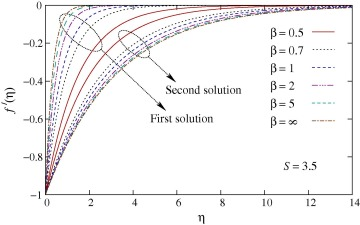Figure 1. Effect of β on the velocity profiles f′(η).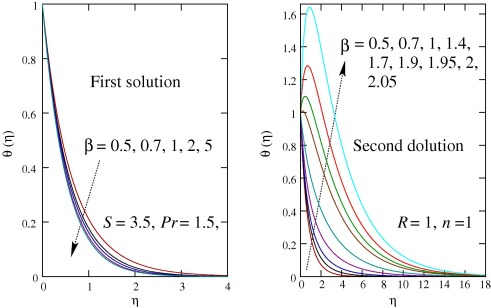Figure 2. Effect of β on the temperature profiles θ(η).

The dimensionless velocity and temperature profiles for various values of wall mass transfer parameter are depicted in Figs. 3 and 4, respectively. Similar to that of Casson parameter, it is observed that for increasing mass suction (S > 0) the velocity and thermal boundary layer thicknesses reduce for first solution and those become thicker for second solution. For stronger mass suction temperature overshoot is obtained in second solution only.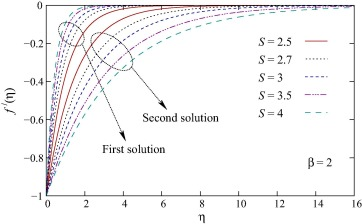Figure 3. Effect of S on the velocity profiles f′(η).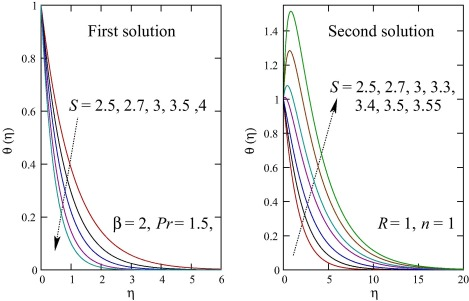Figure 4. Effect of S on the temperature profiles θ(η).

In Fig. 5, the temperature profiles for various values of the Prandtl number Pr and the radiation parameter R are plotted. Due to increase in the Prandtl number the temperature at a point decreases and the thermal boundary layer thickness reduces. Similar effects are noticed for the variation in R. In both cases, it is worth noting that for smaller values of Pr and R thermal overshoots are found in second solution.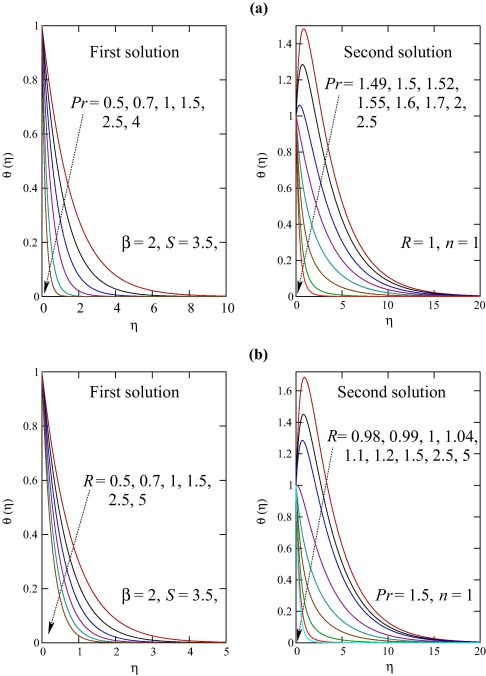Figure 5. (a) Effect of Pr and (b) Effect of R on the temperature profiles θ(η).

The variations in dimensionless temperature profiles for different values of power-law index n are exhibited in Fig. 6. When the wall temperature directly varies with the distance along the sheet, i.e., when n > 0 the temperature in the flow field increases with n for both solutions. On the other hand, for inverse variation of wall temperature (n < 0) with the distance along the sheet, the temperature decreases with the increasing magnitude of n. More importantly, thermal overshoot is viewed in some situations for direct variation (n > 0) in first solution (for large n) and in addition, for second solution the overshoot is always found (even for small n). The thermal overshoot implies that there heat transfers from the ambient fluid to the surface, i.e., heat absorption.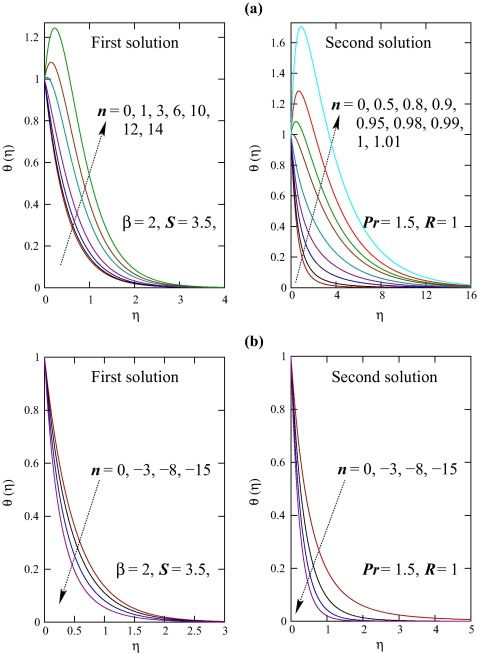Figure 6. Effect of n (a. n positive and b. n negative) on the temperature profiles θ(η).

The quantities f″(0) and −θ′(0) related to the local skin friction coefficient and wall temperature gradient (rate of heat transfer) respectively are plotted in Figure 7, Figure 8, Figure 9, Figure 10, Figure 11 and Figure 12 and also presented in Table 1 and Table 2 for several values involved parameters. The value of f″(0) increases with Casson parameter β and wall mass transfer parameter S for first solution and it decreases for both parameter in second solution ( Fig. 7). Consequently, the local skin friction coefficient increases (decreases) for first (second) solution. The quantity −θ′(0) related to the wall temperature gradient increases with Casson parameter, wall mass transfer parameter, Prandtl number and radiation parameter, which implies that the rate of heat transfer increases. For some values of wall mass transfer parameter the values of −θ′(0) are negative, which means heat absorption occurs in some situations. The heat absorption is found for higher values β and smaller values of Pr and R. Whereas, when β, Pr and R (2, 1.5 and 1 respectively) are fixed, the heat absorption is observed when n ⩾ 1 (approximately). Also, the values of −θ′(0) decrease with increasing values of n (>0, i.e., for direct variation) and the reduction is very prominent for second solution while minor effect is found for first solution. For inverse variation, i.e., for n < 0 the value of −θ′(0) increases with the magnitude of n. In addition to the abovementioned figures, two tables ( Table 1 and Table 2) are presented to show the effects and to demonstrate the current results.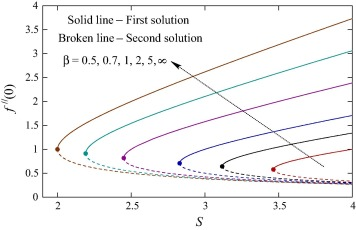Figure 7. Values of f″(0) against S for various values of β.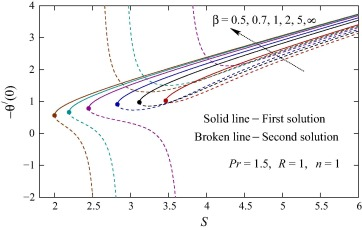Figure 8. Values of −θ′(0) against S for various values of β.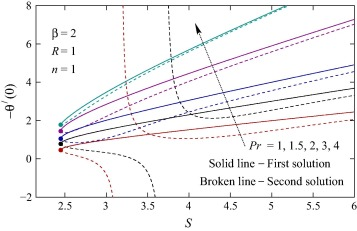Figure 9. Values of −θ′(0) against S for various values of Pr.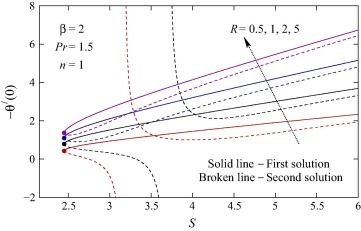Figure 10. Values of −θ′(0) against S for various values of R.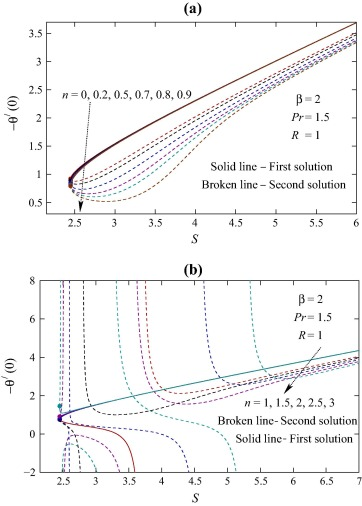Figure 11. Values of −θ′(0) against S for various values of n(>0).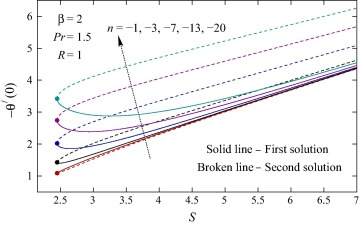Figure 12. Values of −θ′(0) against S for various values of n(<0).

Table 1. Values of f″(0) for various values of β and S.
β S 3 3.5 4 4.5 5 5.5 6
0.5 1st 0.66667 1.00000 1.22871 1.43426 1.62867 1.81650
2nd 0.50000 0.33333 0.27129 0.23241 0.20467 0.18350
1 1st 1.00000 1.39039 1.70711 2.00000 2.28078 2.55425 2.82288
2nd 0.50000 0.35961 0.29289 0.25000 0.21922 0.19575 0.17712
2 1st 1.57735 2.00000 2.38743 2.75831 3.11963 3.47481 3.82574
2nd 0.42265 0.33333 0.27924 0.24169 0.21370 0.19186 0.17426
5 1st 2.10391 2.59561 3.06110 3.51277 3.95602 4.39367 4.82737
2nd 0.39609 0.32105 0.27223 0.23723 0.21065 0.18967 0.17263
1st 2.61803 3.18614 3.73205 4.26556 4.79129 5.31174 5.82843
2nd 0.38197 0.31386 0.26795 0.23444 0.20871 0.18826 0.17157

Table 2. Values of −θ′(0) for various values of β, Pr, R, n, and S.
β Pr R n S 3 3.5 4 4.5 5 5.5 6
0.5 1.5 1 1 1st 1.15415 1.78223 2.23840 2.64831 3.03443 3.40597
2nd 0.96380 1.31949 1.82664 2.29774 2.72924 3.13457
1 1st 1.27345 1.77480 2.18333 2.56113 2.92315 3.27560 3.62169
2nd 0.73385 0.95647 1.47881 2.00401 2.45817 2.87018 3.25974
2 1st 1.52040 1.92819 2.30189 2.65958 3.00804 3.35056 3.68898
2nd 0.32261 −0.70403 2.40233 2.18650 2.54154 2.93656 3.31900
5 1st 1.61279 1.99439 2.35484 2.70420 3.04682 3.38496 3.71995
2nd 3.22798 1.50380 1.73848 2.15325 2.57610 2.97424 3.35358
1st 1.66269 2.03187 2.38537 2.73016 3.06950 3.40514 3.73817
2nd 1.38753 1.37235 1.73036 2.17636 2.60288 2.99914 3.37629
2 1 1st 0.99025 1.26781 1.52010 1.76066 1.99449 2.22400 2.45053
2nd −0.80381 1.33692 1.06088 1.20424 1.47168 1.77028 2.05714
2 1st 2.01310 2.55825 3.05796 3.53609 4.00169 4.45919 4.91110
2nd 1.06965 1.76075 2.44503 3.02919 3.56267 4.06967 4.56004
3 1st 2.84541 3.68883 4.45800 5.19025 5.90053 6.59641 7.28221
2nd 2.21600 3.18540 4.04281 4.83436 5.58781 6.31684 7.02906
4 1st 3.47592 4.63497 5.69264 6.69557 7.66446 8.61041 9.54002
2nd 3.28267 4.50579 5.59699 6.61968 7.60148 8.55648 9.49280
1.5 0.5 1st 0.94112 1.20701 1.44838 1.67838 1.90185 2.12113 2.33754
2nd −0.87748 1.28930 1.00366 1.12463 1.37276 1.66002 1.93920
2 1st 2.10581 2.67945 3.20506 3.70779 4.19721 4.67803 5.15289
2nd 1.19420 1.92145 2.61176 3.21212 3.76638 4.29513 4.80745
5 1st 2.66733 3.43777 4.14111 4.81156 5.46258 6.10093 6.73044
2nd 1.95648 2.86601 3.67156 4.40980 5.10981 5.78567 6.44502
1 0 1st 1.54501 1.94247 2.31174 2.66703 3.01401 3.35553 3.69323
2nd 1.17786 1.60393 2.02243 2.41719 2.79388 3.15840 3.51452
0.5 1st 1.53090 1.93439 2.30624 2.66293 3.01076 3.35285 3.69096
2nd 0.91515 1.35857 1.83537 2.27052 2.67138 3.05198 3.41989
0.8 1st 1.52413 1.93044 2.30349 2.66083 3.00907 3.35143 3.68974
2nd 0.66089 1.06784 1.65305 2.16096 2.58978 2.98369 3.36019
1.5 1st 1.51406 1.92405 2.29874 2.65702 3.00586 3.34866 3.68728
2nd 1.21697 1.03657 1.36012 1.86543 2.36534 2.80620 3.21008
2 1st 1.51255 1.92215 2.29688 2.65527 3.00424 3.34716 3.68589
2nd 0.39617 0.25604 −0.01527 −5.69389 2.68637 2.70790 3.09406
2.5 1st 1.51663 1.92270 2.29638 2.65438 3.00321 3.34608 3.68481
2nd −0.33990 −8.39716 1.86128 1.58297 1.94817 2.47395 2.95479
−1 1st 1.58224 1.96368 2.32588 2.67735 3.02200 3.36198 3.69862
2nd 1.53217 1.92956 2.30223 2.65980 3.00822 3.35071 3.68911
−3 1st 1.68316 2.02275 2.36521 2.70566 3.04353 3.37903 3.71255
2nd 2.02040 2.38722 2.72287 3.04648 3.36491 3.68109 3.99638
−7 1st 1.94372 2.18707 2.47789 2.78750 3.10559 3.42774 3.75187
2nd 2.69807 3.03843 3.34503 3.63955 3.92964 4.21866 4.50821
−13 1st 2.38533 2.49594 2.70212 2.95568 3.23538 3.53043 3.83488
2nd 3.43725 3.76094 4.04923 4.32443 4.59458 4.86339 5.13272
−20 1st 2.90145 2.89304 3.00878 3.19541 3.42562 3.68375 3.96031
2nd 4.11493 4.42893 4.70643 4.96992 5.22765 5.48348 5.73944

## 6. Conclusions

The heat transfer in boundary layer flow of Casson fluid past a shrinking sheet with variable wall temperature and thermal radiation effect is investigated. The transformed self-similar nonlinear ordinary differential equations are obtained and then those are solved analytically. It is found that the velocity increases (decreases) with Casson parameter and wall mass transfer parameter for first (second) solution and the effect is opposite for the temperature. Consequently, the thermal boundary layer thickness decreases with Casson parameter and wall mass transfer parameter for first solution and it increases for second solution. On the other hand, the thermal boundary layer thickness reduces with the Prandtl number and the radiation parameter for both solutions. The local skin friction coefficient increases with Casson parameter and wall mass transfer parameter for first solution and the effect is reverse for second solution. The rate of heat transfer decreases with increasing values of power-law exponent for direct variation along the sheet and for inverse variation it increases. Importantly, heat absorption is found for second solution and in certain cases for first solution.

## Acknowledgements

The authors express their sincere thanks to the esteemed referees for their constructive suggestions. One of the authors, K. Bhattacharyya gratefully acknowledges the financial support of National Board for Higher Mathematics (NBHM), Department of Atomic Energy, Government of India, for pursuing this work. G.C. Layek would like to acknowledge the assistance and support received from UGC, DSA-I in the Department of Mathematics, The University of Burdwan.

### Document informationPublished on 12/04/17

Licence: Other

### Document Score0

Views 15
Recommendations 0